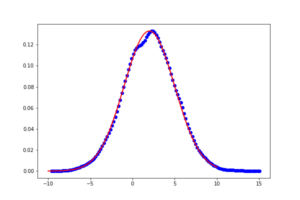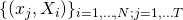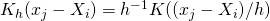# Nonparametric Density estimation using Spark

## 1. A Nonparametric Density implementation in SparkThe red curve shows the true density while the blue dots show the estimated density evaluated using an equidistant grid.One of my previous blog post concerns about nonparametric density estimation. In this post i presented some Matlab code. An advantage of this Spark implementation is that the estimation is totally parallel since we only use build-in Spark procedures. Letbe a random sample drawn from some distribution with an unknown density. The key is to use data.cartesian(random_grid) which creates pairswhereis a predefined grid. Then using map together with an Epanechnikov kernelwe get. The finalis then evaluated using reduceByKey.

###A Spark-Function to derive a non-parametric kernel density

from pyspark.mllib.regression import LabeledPoint
from pyspark.mllib.feature import StandardScaler
import matplotlib.pyplot as plt
from numpy import *

##1.0 Simulated Data
N=15000
mu, sigma = 2, 3 # mean and standard deviation
rdd = sc.parallelize( random.normal(mu,sigma,N) )

##2.0 The Function
#2.1 Kernel Function

def spark_density(data, Nout, bw):
def epan_kernel(x,y,b):
u=true_divide( (x-y), b)
return max(0, true_divide( 1, b)*true_divide(3,4)*(1-u**2))

#derive the minia and maxi used for interpolation
mini=data.takeOrdered(1, lambda x: x )
maxi=data.takeOrdered(1, lambda x: -1*x )
#create an interpolation grid (in fact NOT random this time)
random_grid = sc.parallelize( linspace(mini, maxi, num=Nout)   )
Nin=data.count()
#compute K(x-xi) Matrix
kernl=data.cartesian(random_grid).map(lambda x:( float(x),true_divide(epan_kernel(array(x),array(x),bw),Nin) ) )
#sum up
return kernl.reduceByKey( lambda y, x:  y+x )

##3.0 Results

density= spark_density(rdd, 128, 0.8).collect()
dens=array(density).transpose()

anzahl=array(anz).transpose()
#Plot the estimate
plt.plot(dens, dens, 'bo')

axis2=linspace(-10, 10, num=128)
#plot the true density
plt.plot(axis2, 1/(sigma * sqrt(2 * pi)) *exp( - (axis2 - mu)**2 / (2 * sigma**2) ),linewidth=2, color='r')
plt.show()


### 4 thoughts on “Nonparametric Density estimation using Spark”

1.Stupid question: can i just use R density for it?
•